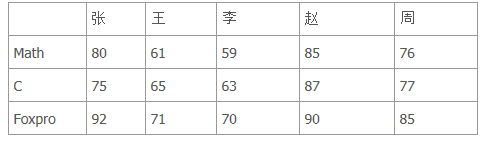# C语言二维数组的定义和引用

7.1.1二维数组的定义

int a;

a,a,a,a

a,a,a,a

a,a,a,a

7.1.2二维数组元素的引用

a

【例7.6】一个学习小组有5个人，每个人有三门课的考试成绩。求全组分科的平均成绩和各科总平均成绩。main()

{

int i,j,s=0,average,v,a;

printf("input score\n");

for(i=0;i<3;i++)

{

for(j=0;j<5;j++)

{ scanf("%d",&a[j][i]);

s=s+a[j][i];}

v[i]=s/5;

s=0;

}

average =(v+v+v)/3;

printf("math:%d\nc languag:%d\ndbase:%d\n",v,v,v);

printf("total:%d\n", average );

}

7.1.3二维数组的初始化

int a={ {80,75,92},{61,65,71},{59,63,70},{85,87,90},{76,77,85} };

int a={ 80,75,92,61,65,71,59,63,70,85,87,90,76,77,85};

【例7.7】

main()

{

int i,j,s=0, average,v;

int a={{80,75,92},{61,65,71},{59,63,70},{85,87,90},{76,77,85}};

for(i=0;i<3;i++)

{ for(j=0;j<5;j++)

s=s+a[j][i];

v[i]=s/5;

s=0;

}

average=(v+v+v)/3;

printf("math:%d\nc languag:%d\ndFoxpro:%d\n",v,v,v);

printf("total:%d\n", average);

}

int a={{1},{2},{3}};

1 0 0

2 0 0

3 0 0

int a ={{0,1},{0,0,2},{3}};

0 1 0

0 0 2

3 0 0

int a={1,2,3,4,5,6,7,8,9};

int a[]={1,2,3,4,5,6,7,8,9};

a

a

a

JavaEE